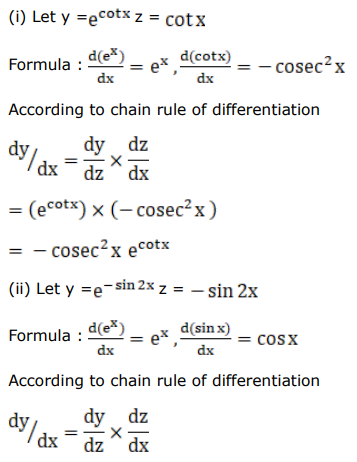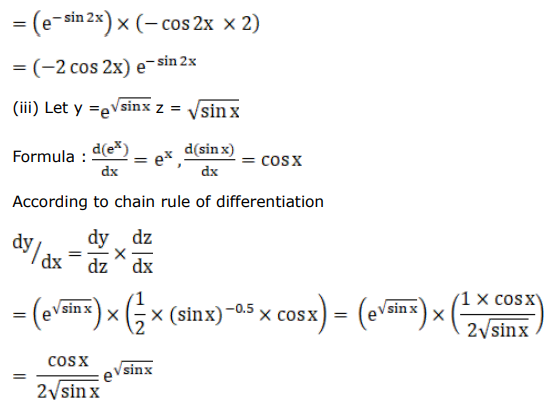# Differentiate each of the following w.r.t.Question:

Differentiate each of the following w.r.t. $x$ :

(i) $e^{\cot x}$

(ii) $e^{-\sin 2 x}$

(iii) $e^{\sqrt{\sin x}}$

Solution: